#Qualitative Analysis of Linear SystemsIn this page, we will summarize the behavior of the solutions of linear systems. First consider the linear system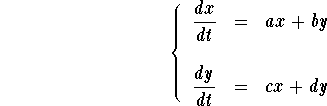The associated eigenvalues are the roots of the characteristic polynomial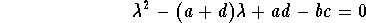Depending on the eigenvalues, the solutions have different behavior.Two Real nonzero eigenvalues. We have three cases:The two eigenvalues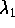and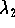are positive (with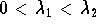). When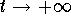, the solutions explode tangent to the straight-line solution associated to the eigenvalue.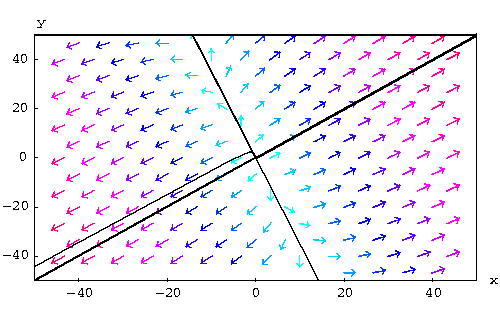In this case the equilibrium point is a source.The two eigenvaluesandare negative (with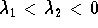). When, the solutions "die" at the origin. They tend to the equilibrium point tangent to the straight-line solution associated to the eigenvalue.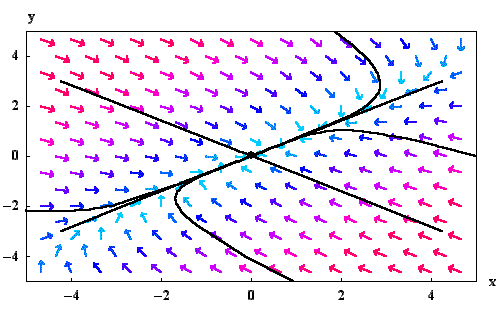In this case the equilibrium point is a sink.The two eigenvaluesandhave different signs (with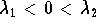). In this case, the solutions explode whether when(except along the straight-line solution associated to the eigenvalue) or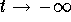(except along the straight-line solution associated to the eigenvalue).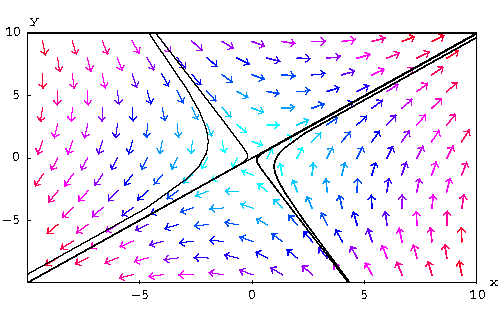In this case, the equilibrium point is a saddle.Repeated Real nonzero eigenvalue. Let us call this eigenvalue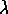. We have two casesIf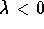, then the solutions tend either to the equilibrium point tangent to the only straight-line solution,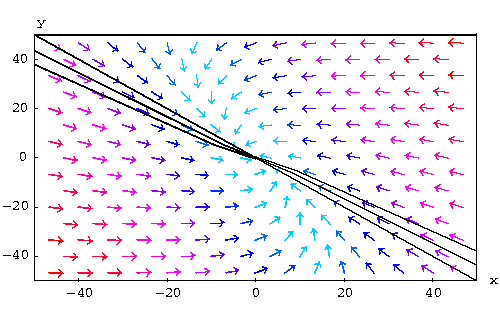or it can happen that all solutions (except for the equilibrium point) are straight-line solutions, approaching the equilibrium point: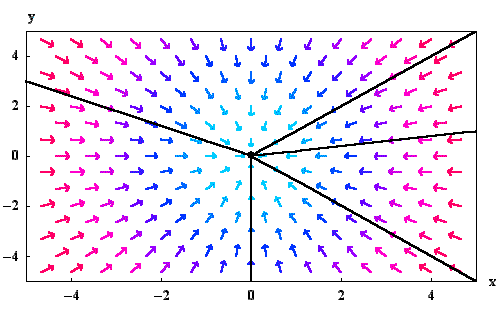If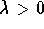, then the solutions get large as. But even if the solution explodes, it does go to infinity either tangent to the straight-line solution,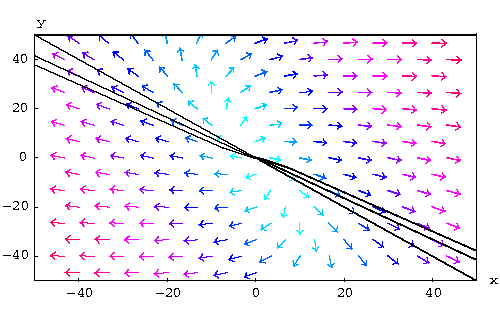or goes to infinity straight in every direction: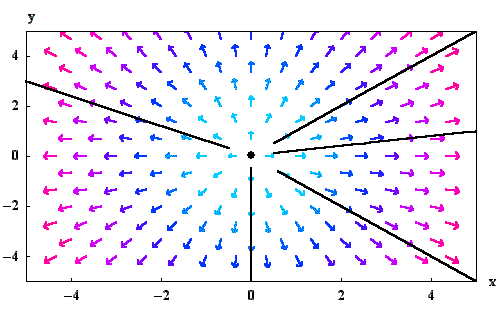Zero eigenvalue. If the system has zero as an eigenvalue, then there exists a line of equilibrium points (degenerate case). Let us call the other eigenvalue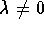. Note that the solutions are all straight-line solutions. Depending on the sign of, the solution may tend to or get away from the line of equilibrium points parallel to the eigenvector associated to the eigenvalue. For a negative, we have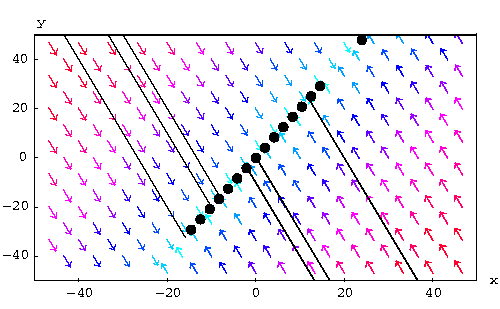and for a positive, we have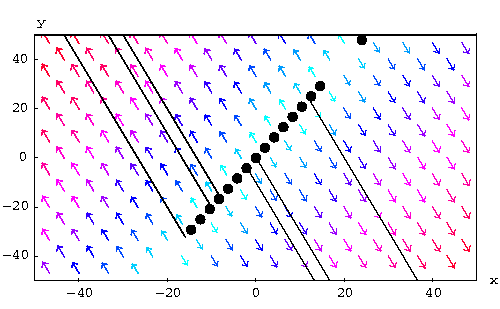Complex eigenvalues. Let us write the eigenvalues as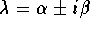. We have three cases.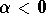. The solutions tend to the origin (when) while spiraling. In this case, the equilibrium point is called a spiral sink.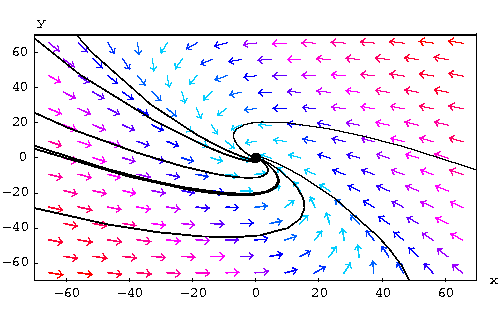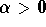. The solutions explode or get away from the origin (when) while spiraling. In this case, the equilibrium point is called a spiral source.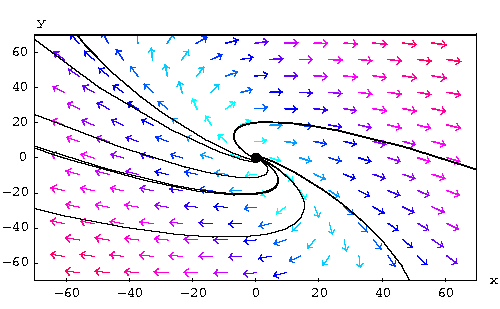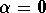. The solutions are periodic. This means that the trajectories are closed curves or cycles. In this case, the equilibrium point is called a center.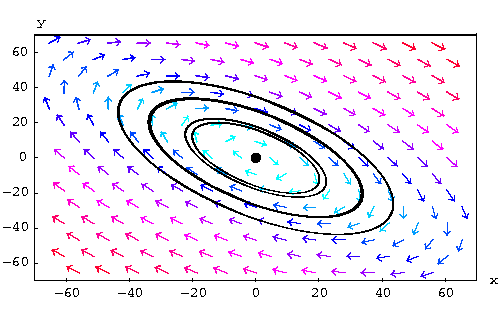[Differential Equations] [First Order D.E.]
[Geometry] [Algebra] [Trigonometry ]
[Calculus] [Complex Variables] [Matrix Algebra]S.O.S MATHematics home page

Do you need more help? Please post your question on our S.O.S. Mathematics CyberBoard.Author: Mohamed Amine Khamsi